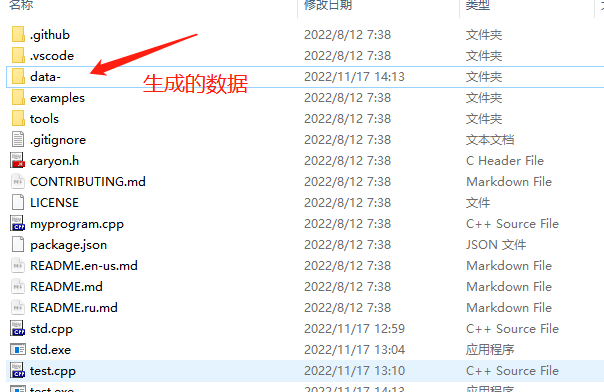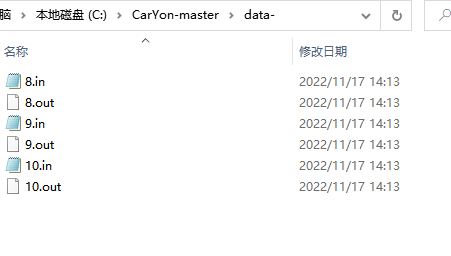# CarYon简明使用指南

github

wiki下载

## 02_解压缩

• examples
• A+B Problem
• CF1A Theatre square
• Luogu P1093
• Luogu P3366
• tools
• Compare
• Configurstion-File-Analysis
• Extensions
• caryon.h
• myprogram.cpp
• test.cpp
• std.cpp

### 简要说明

• example

这个文件夹会存放一些使用的例子:

• A+B Problem : 最基础的样例生成
• CF1A ：较为基础的样例生成
• P1093：多组样例生成
• P3366 ：图论相关的数据生成
• tools

相关工具。初级使用时不用关注。

• Compare：对拍器
• Configurstion-File-Analysis：仅编写配置文件生成数据
• Extension：插件

## 03_制作数据

### 03_2 test文件

#include "caryon.h"
// including <bits/stdc++.h>
using namespace std;
using namespace ca;  // namespace
int main() {
dataname = "";  // dataname
maxtime  = 1000;
makein(/*start*/, /*end*/) {
csh();
}
makeout(/*start*/, /*end*/);
//debug(/*start*/, /*end*/); 程序对拍
return 0;
}

1. datename 中存放题目名称。之后会生成 name1.inname1.out 这样对应的数据。建议留空,使其生成1.in1.out 这样的数据。
2. maxtime：程序实现。不用改动。
3. makeinmakeout 中的startend参数。对应你需要生成的数据点开始、结束编号。如你想生成start.in,start.out ~ end.in,end.out 的数据，填上相应的参数即可。
4. makein 内部,csh 的下方，根据输入要求书写对应的代码。

### 03_3 随机数据

cyrand(a,b) 可返回一个在 ab之间的随机数。

### 03_4 写入in文件

• inint(int) ：将一个整型的变量或常量写入每一个输入文件。
• instring(std::string) ：将一个 C++ 字符串写入每一个输入文件。
• 任意类型格式：in<元素类型>(对应类型数据) : 例：long long a;in<long long>(a)
• inendl()：在输入文件中打印一个换行符。
• inspace()：在输入文件中打印一个空格。

## 例子说明

$1$ 行一个整数 $N$

$2$$N+1$ 行，第 $i+1$ 行有两个整数 $H_i$$V_i$，表示第 $i$ 个人的高度和发射的能量值。

#include "caryon.h"
// including <bits/stdc++.h>
using namespace std;
using namespace ca;  // namespace
int main() {
dataname = "";  // dataname留空
maxtime  = 1000;
makein(/*start*/8, /*end*/10) {//针对100%的数据，生成测试点8.in ~ 测试点10.in
csh();
int n=cyrand(1e5,1e6);// n的范围 1e5 ~ 1e6 ，与前面的70%的数据作区分。
inint(n);//写入n
instring("\n");//写入换行 也可用inendl()
for(int i=1;i<=n;i++){//循环n次
inint(cyrand(1,2e9));//写入随机出的Hi, 范围 1 ~ 1e9
instring(" ");//写入空格
inint(cyrand(1,1e3));//写入随机出的Vi, 范围 1 ~ 1e3
instring("\n");//写入换行
}
}
makeout(/*start*/8, /*end*/10);//针对100%的数据，生成测试点8.out ~ 测试点10.out
//debug(/*start*/, /*end*/);
return 0;
}